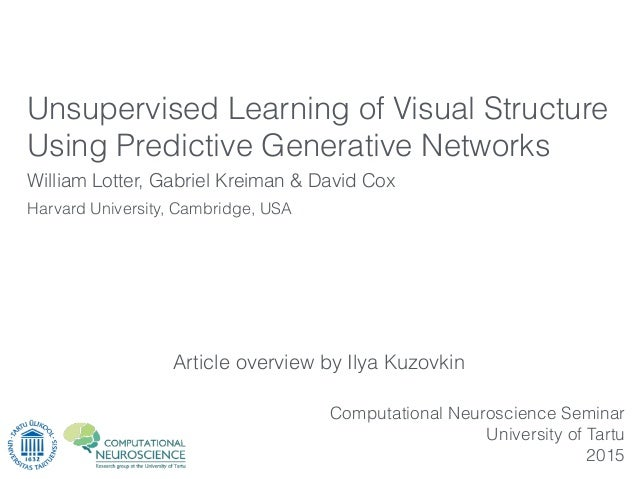Successfully reported this slideshow.
We use your LinkedIn profile and activity data to personalize ads and to show you more relevant ads. You can change your ad preferences anytime.Upcoming SlideShare
Loading in …5
×

# Article overview: Unsupervised Learning of Visual Structure Using Predictive Generative Networks

This set of slides goes over the recent article that tries to tie together the idea of predictive coding and deep learning. The main point of the article is that a generative system trained on sequential data to predict the future samples learns more "useful" representation than the usual autoencoder. The result resonates with the fact that our brain is probably using predictive mechanisms.

• Full Name
Comment goes here.

Are you sure you want to Yes No
Your message goes here• Be the first to comment

### Article overview: Unsupervised Learning of Visual Structure Using Predictive Generative Networks

1. 1. Article overview by Ilya Kuzovkin William Lotter, Gabriel Kreiman & David Cox Computational Neuroscience Seminar University of Tartu 2015 Harvard University, Cambridge, USA Unsupervised Learning of Visual Structure Using Predictive Generative Networks
2. 2. The idea of predictive coding in neuroscience
3. 3. “state-of-the-art deep learning models rely on millions of labeled training examples to learn”
4. 4. “state-of-the-art deep learning models rely on millions of labeled training examples to learn” “in contrast to biological systems, where learning is largely unsupervised”
5. 5. “state-of-the-art deep learning models rely on millions of labeled training examples to learn” “in contrast to biological systems, where learning is largely unsupervised” “we explore the idea that prediction is not only a useful end-goal, but may also serve as a powerful unsupervised learning signal”
6. 6. PART I THE IDEA OF PREDICTIVE ENCODER "prediction may also serve as a powerful unsupervised learning signal"
7. 7. PREDICTIVE GENERATIVE NETWORK (a.k.a “Predictive Encoder” Palm 2012) vs.
8. 8. input output “bottleneck” AUTOENCODER
9. 9. input output “bottleneck” AUTOENCODER
10. 10. input output “bottleneck” AUTOENCODER
11. 11. input output “bottleneck” Reconstruction AUTOENCODER
12. 12. input output “bottleneck” Reconstruction AUTOENCODER
13. 13. input output “bottleneck” Can we do prediction? Reconstruction AUTOENCODER
14. 14. PREDICTIVE GENERATIVE NETWORK (a.k.a “Predictive Encoder” Palm 2012) vs.
15. 15. RECURRENT NEURAL NETWORK
16. 16. RECURRENT NEURAL NETWORK
17. 17. RECURRENT NEURAL NETWORK
18. 18. RECURRENT NEURAL NETWORK
19. 19. PREDICTIVE GENERATIVE NETWORK (a.k.a “Predictive Encoder” Palm 2012) vs.
20. 20. PREDICTIVE GENERATIVE NETWORK (a.k.a “Predictive Encoder” Palm 2012) vs. Convolution ReLu Max-pooling 2x {
21. 21. PREDICTIVE GENERATIVE NETWORK (a.k.a “Predictive Encoder” Palm 2012) vs. Long Short-Term Memory (LSTM) 5 - 15 steps 1024 units Convolution ReLu Max-pooling 2x {
22. 22. PREDICTIVE GENERATIVE NETWORK (a.k.a “Predictive Encoder” Palm 2012) vs. Long Short-Term Memory (LSTM) 5 - 15 steps 1024 units Convolution ReLu Max-pooling 2x { 2 layers NN upsampling ReLu Convolution
23. 23. PREDICTIVE GENERATIVE NETWORK (a.k.a “Predictive Encoder” Palm 2012) vs. Long Short-Term Memory (LSTM) 5 - 15 steps 1024 units Convolution ReLu Max-pooling 2x { MSE loss RMSProp optimizer LR 0.001 2 layers NN upsampling ReLu Convolution
24. 24. PREDICTIVE GENERATIVE NETWORK (a.k.a “Predictive Encoder” Palm 2012) vs. Long Short-Term Memory (LSTM) 5 - 15 steps 1024 units http://keras.io 2 layers NN upsampling Convolution ReLu ReLu Convolution Max-pooling 2x { MSE loss RMSProp optimizer LR 0.001
25. 25. PART II ADVERSARIAL LOSS "the generator is trained to maximally confuse the adversarial discriminator"
26. 26. vs. Long Short-Term Memory (LSTM) 5 - 15 steps 1568 units Fully connected layer 2 layers NN upsampling Convolution ReLu ReLu Convolution Max-pooling 2x{ MSE loss RMSProp optimizer LR 0.001
27. 27. vs. Long Short-Term Memory (LSTM) 5 - 15 steps 1568 units Fully connected layer 2 layers NN upsampling Convolution ReLu ReLu Convolution Max-pooling 2x{ MSE loss RMSProp optimizer LR 0.001
28. 28. MSE loss
29. 29. MSE loss
30. 30. MSE loss
31. 31. 3 FC layers (relu, relu, softmax) MSE loss
32. 32. 3 FC layers (relu, relu, softmax) "trained to maximize the probability that a proposed frame came from the ground truth data and minimize it when it is produced by the generator" MSE loss
33. 33. 3 FC layers (relu, relu, softmax) "trained to maximize the probability that a proposed frame came from the ground truth data and minimize it when it is produced by the generator" AL loss to train PGN MSE loss AL loss
34. 34. 3 FC layers (relu, relu, softmax) "trained to maximize the probability that a proposed frame came from the ground truth data and minimize it when it is produced by the generator" AL loss to train PGN MSE loss AL loss
35. 35. “with adversarial loss alone the generator easily found solutions that fooled the discriminator, but did not look anything like the correct samples” MSE model is fairly faithful to the identities of the faces, but produces blurred versions combined AL/MSE model tends to underﬁt the identity towards a more average face
36. 36. PART III INTERNAL REPRESENTATIONS AND LATENT VARIABLES "we are interested in understanding the representations learned by the models"
37. 37. PGN model LSTM activities L2 regression Value of a latent variable
38. 38. PGN model LSTM activities L2 regression Value of a latent variable
39. 39. “An MDS algorithm aims to place each object in N-dimensional space such that the between-object distances are preserved as well as possible.” MULTIDIMENSIONAL SCALING
40. 40. PART IV USEFULNESS OF PREDICTIVE LEARNING "representations trained with a predictive loss outperform other models of comparable complexity in a supervised classiﬁcation problem"
41. 41. THE TASK: 50 randomly generated faces (12 angles per each) Generative models: Internal representation SVM Identify class
42. 42. THE TASK: 50 randomly generated faces (12 angles per each) Generative models: Internal representation SVM Identify class • Encoder-LSTM-Decoder to predict next frame (PGN) • Encoder-LSTM-Decoder to predict last frame (AE LSTM dynamic) • Encoder-LSTM-Decoder on frames made into static movies (AE LSTM static) • Encoder-FC-Decoder with #weights as in LSTM (AE FC #weights) • Encoder-FC-Decoder with #units as in LSTM (AE FC #units)